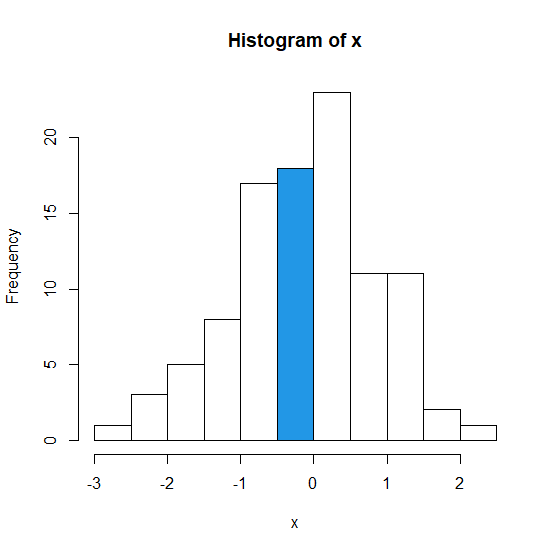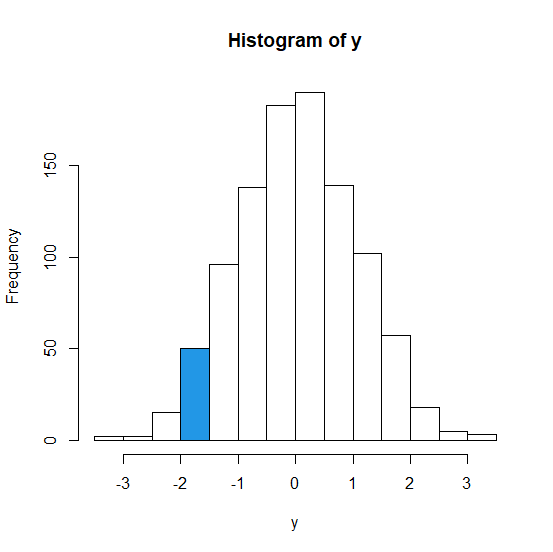# How to highlight a bar in base R histogram?

To highlight a bar in base R histogram, we need to understand the X-axis values and pass the col argument inside hist function appropriately. We just need to put a separate value for the bar that we want to highlight and set the colouring of the rest of the bars to 0 (that is default in base R). Check out the below examples to understand how it works.

## Example1

> x<-rnorm(100)
> hist(x,col = c(rep(0,5),4,rep(0,5)))

## Output## Example2

> y<-rnorm(1000)
> hist(y,col = c(rep(0,3),4,rep(0,9)))

## Output